# RD Sharma Solutions For Class 7 Maths Exercise 4.6 Chapter 4 Rational Numbers

RD Sharma Solutions for Class 7 Exercise 4.6 of Chapter 4 Rational Numbers are provided here. The questions present in this have been solved by BYJU’S experts in Maths, and these solutions guide students to study well and solve lengthy problems in a shorter duration. Learners get the pdf of this chapter from the below-provided links. By exercising RD Sharma Solutions for Class 7 student will understand the concepts perfectly. There are two types of rational numbers i.e. positive and negative. Some of the topics discussed in this exercise are listed below.

• Representation of rational numbers on the number line
• Comparision of rational numbers
• Some useful properties of rational numbers

## Download the PDF of RD Sharma Solutions For Class 7 Maths Chapter 4 – Rational Numbers Exercise 4.6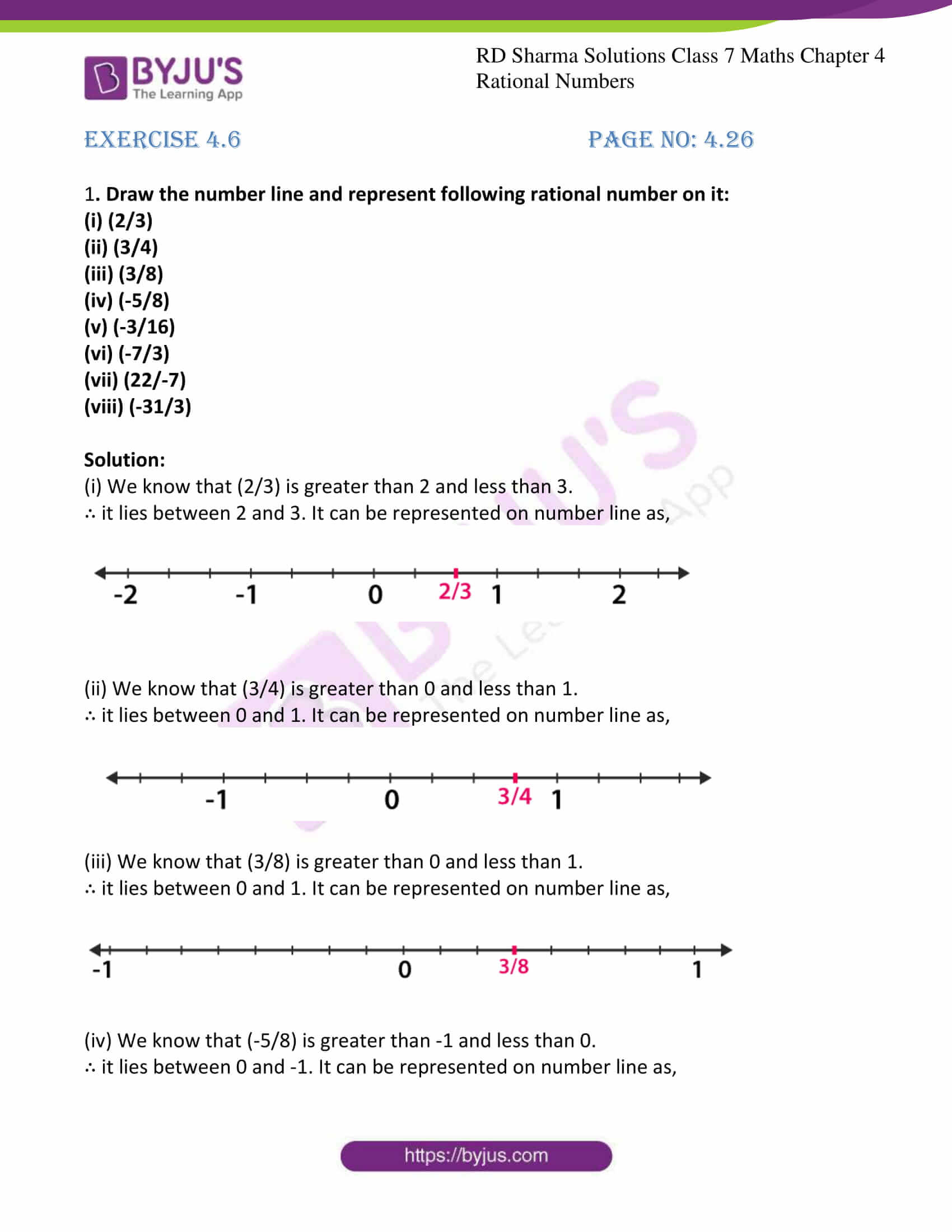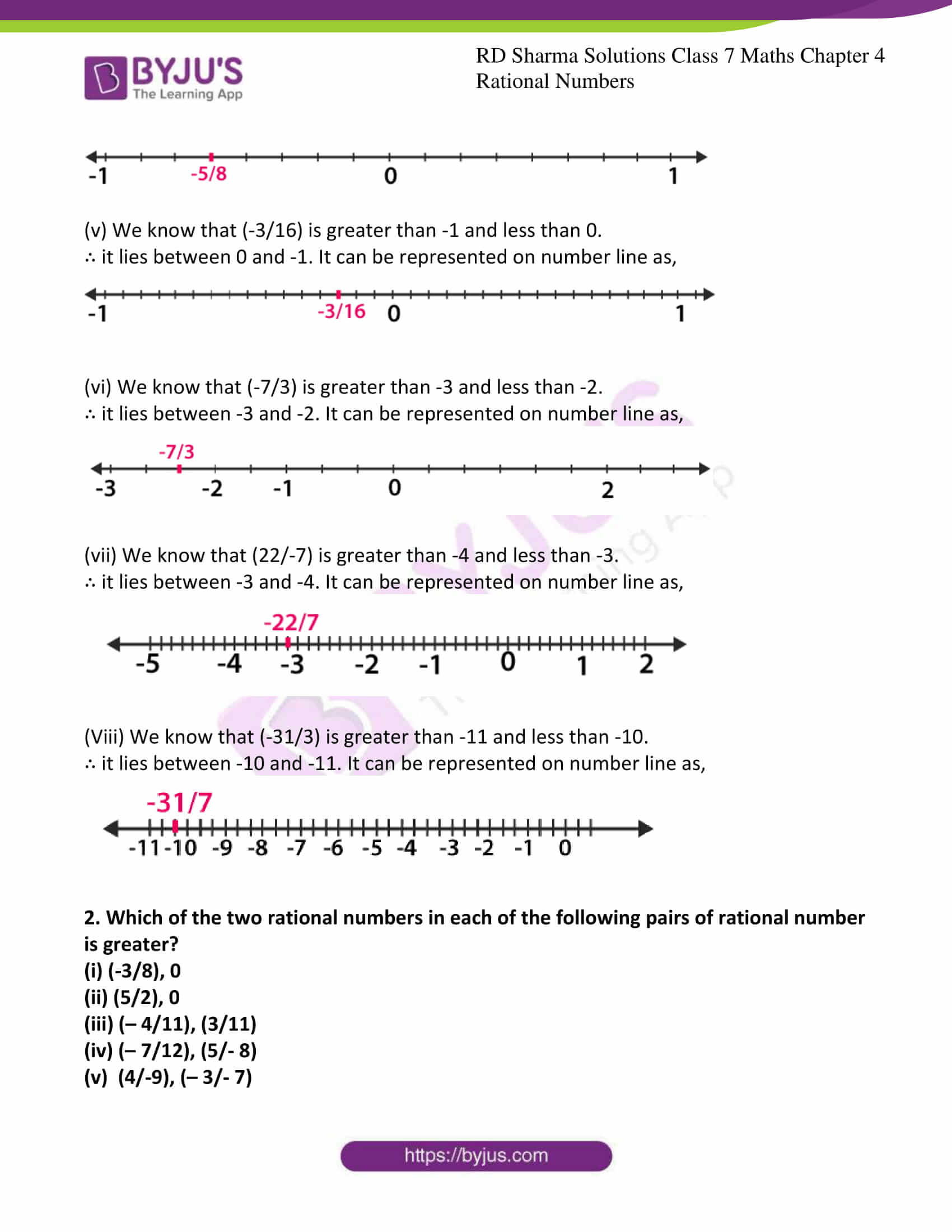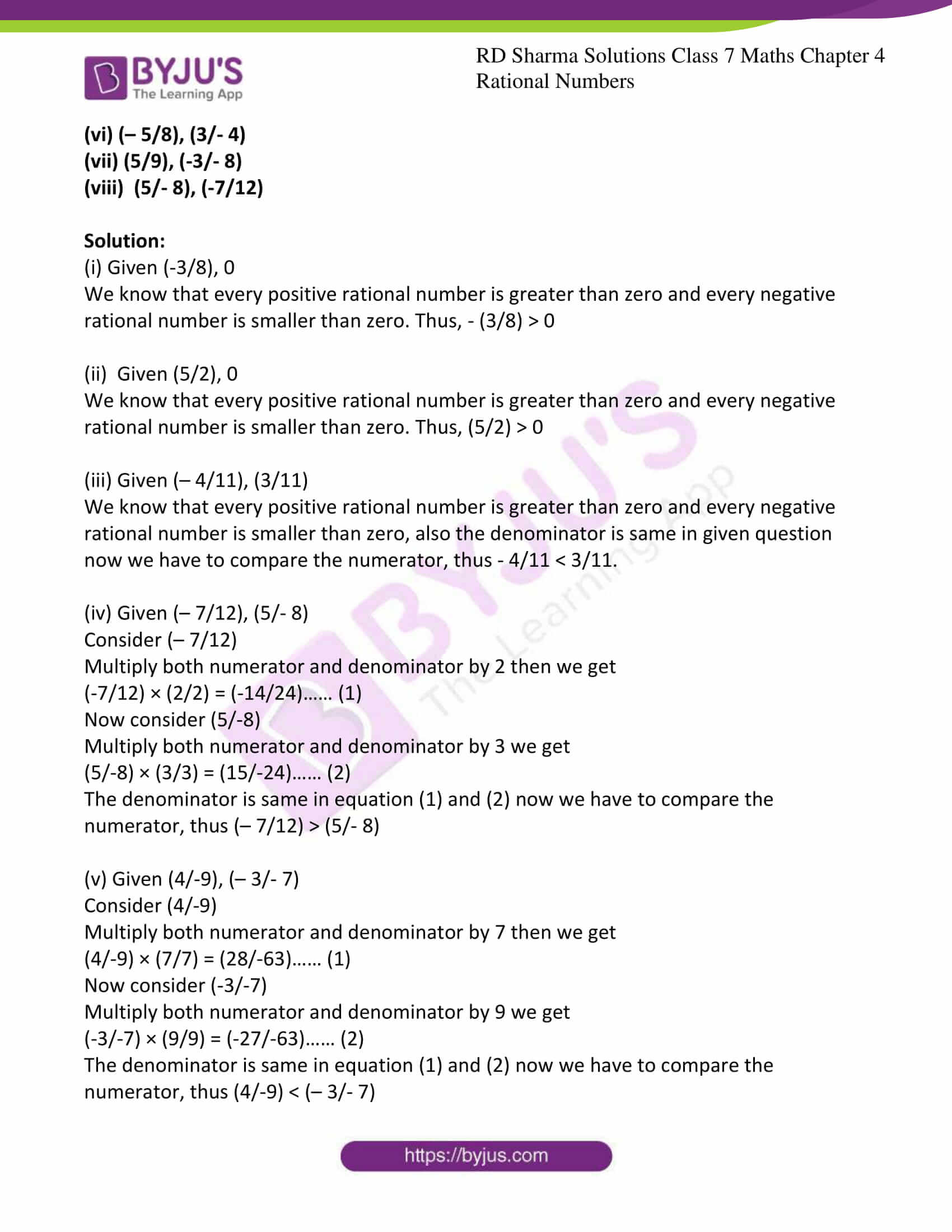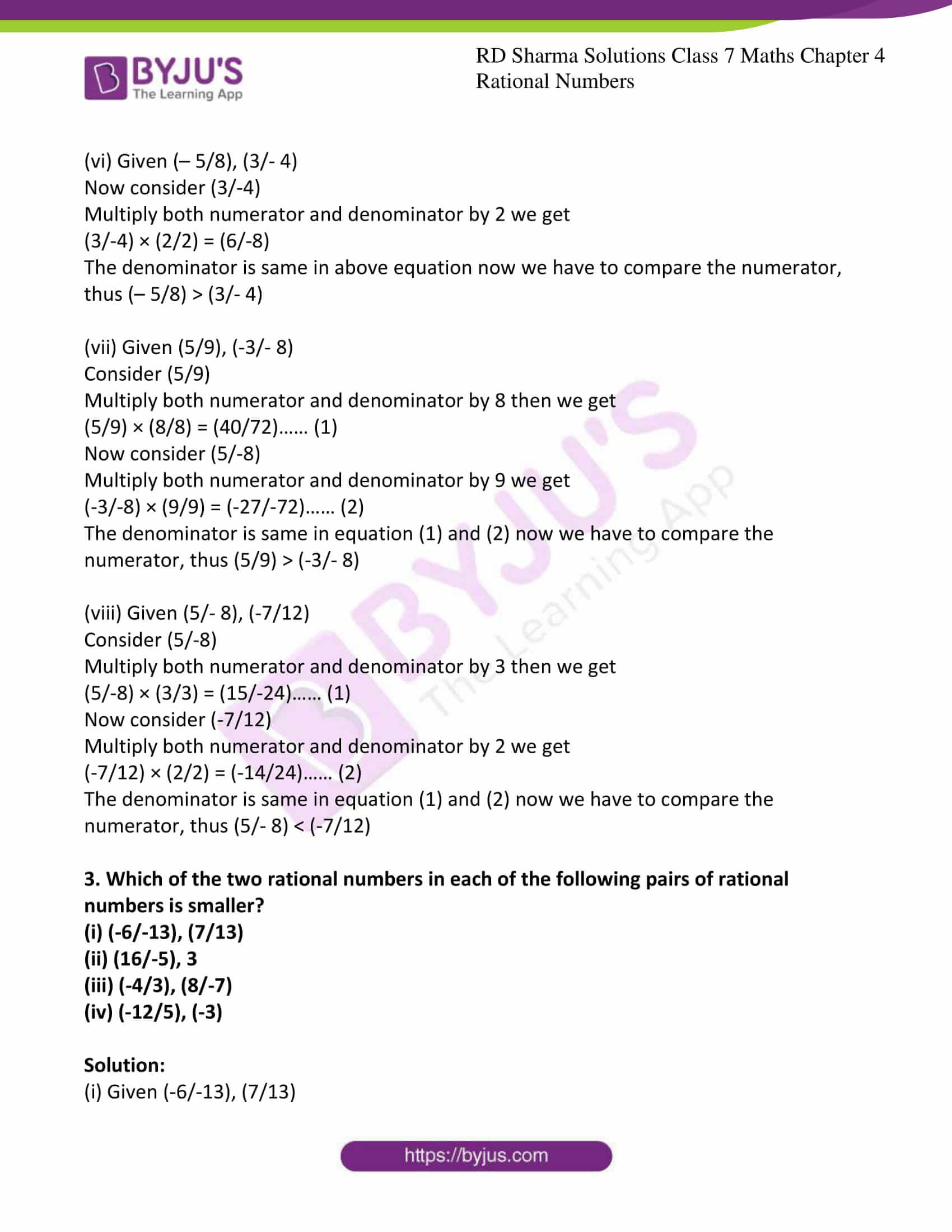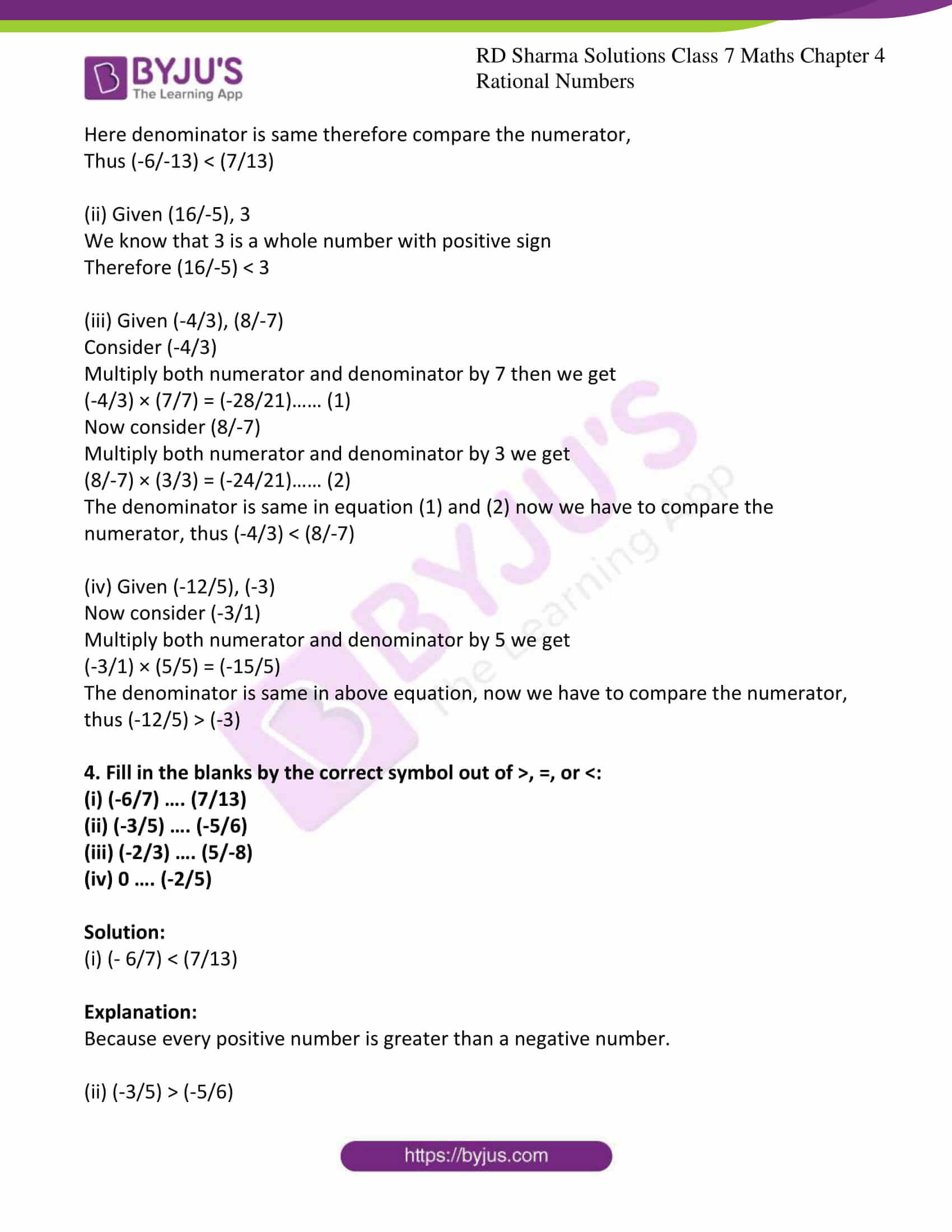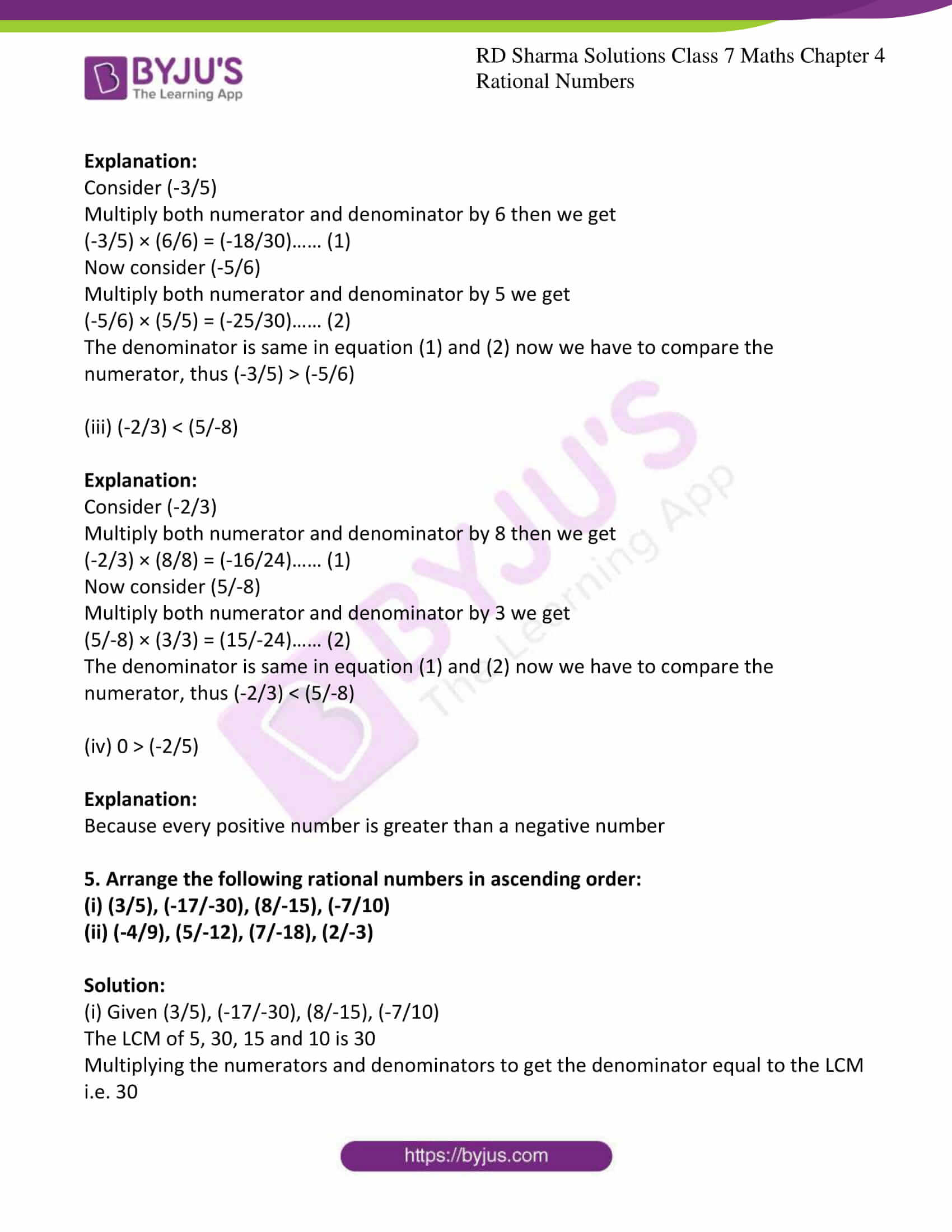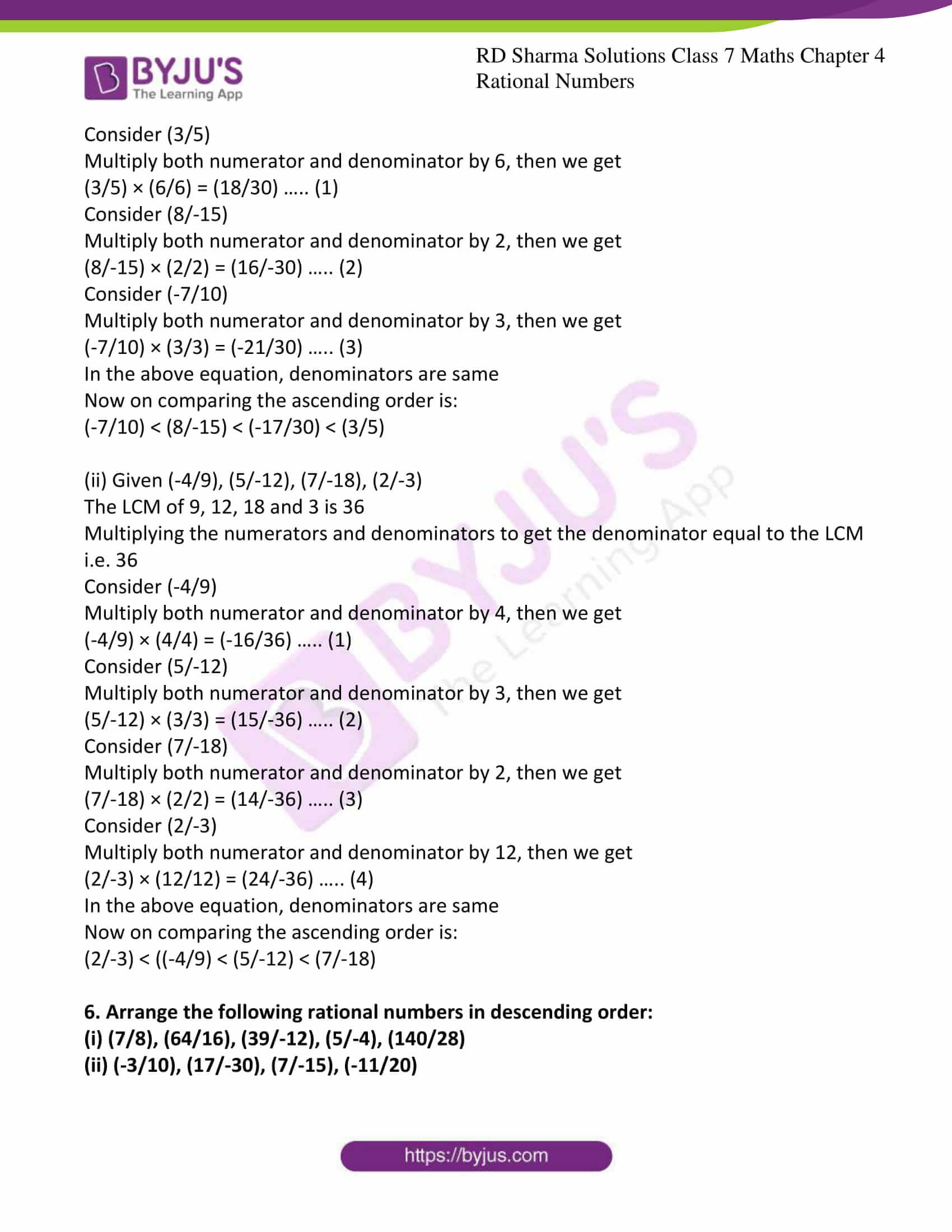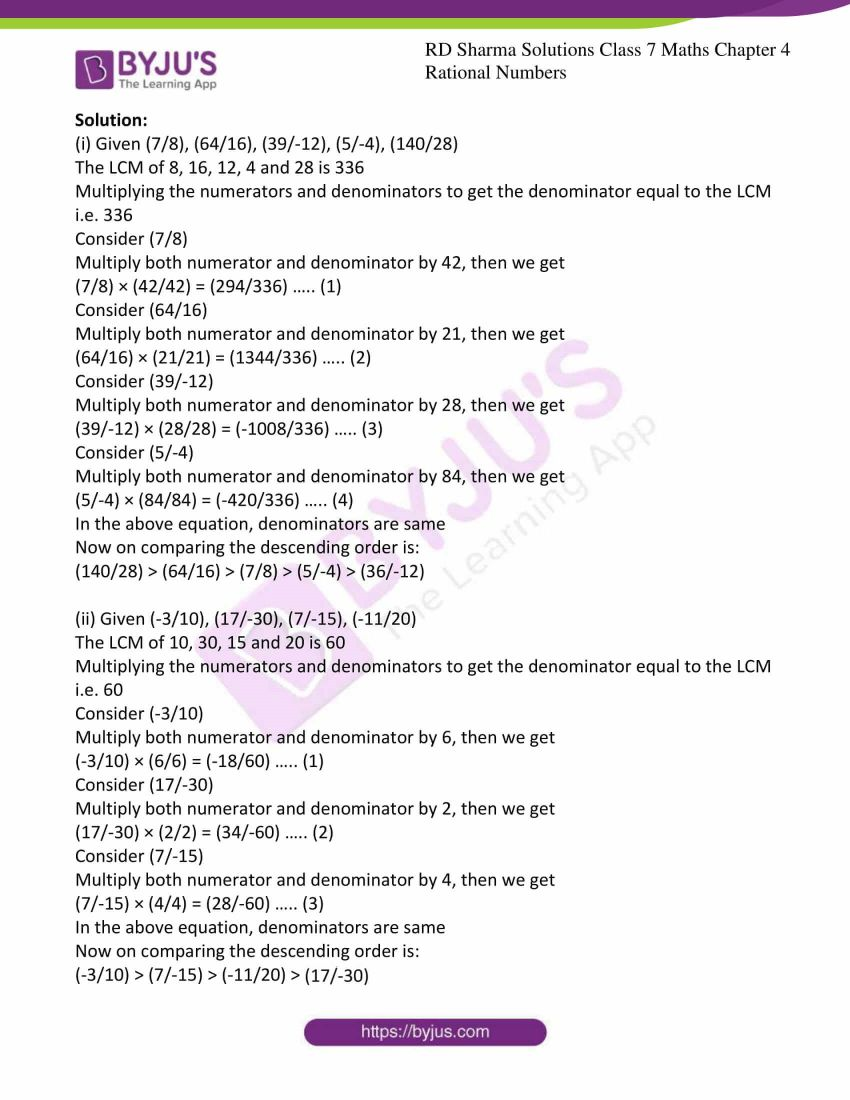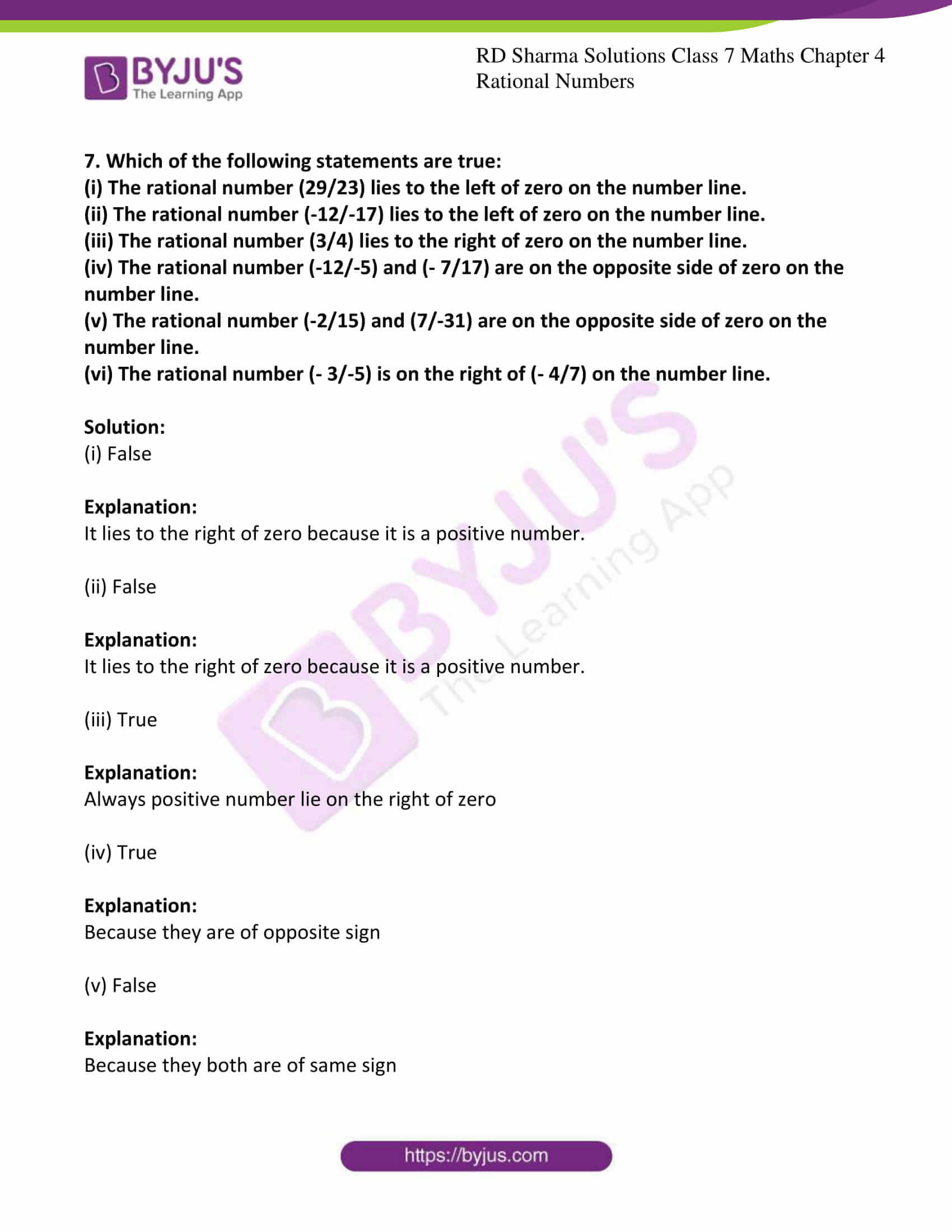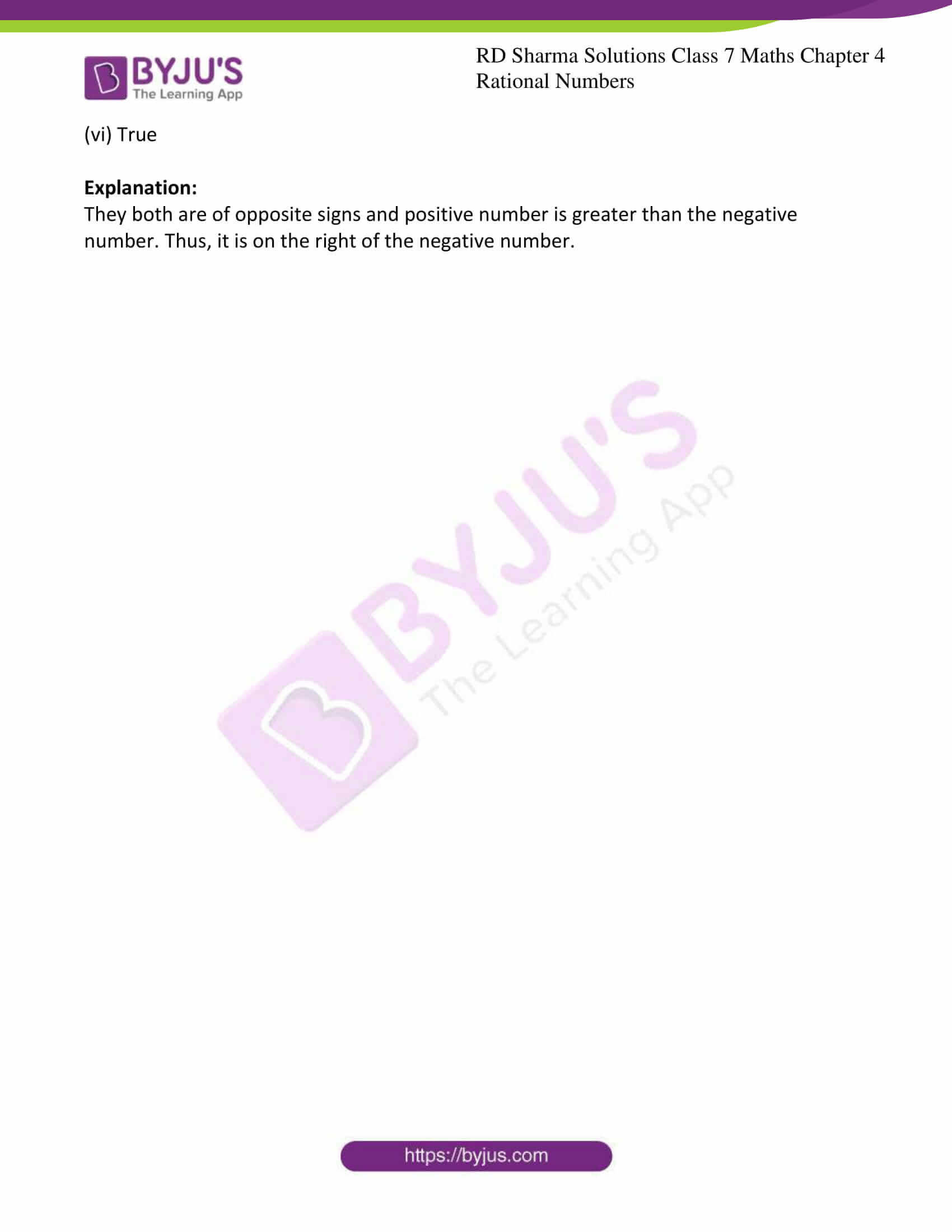### Access answers to Maths RD Sharma Solutions For Class 7 Chapter 4 – Rational Numbers Exercise 4.6

1. Draw the number line and represent following rational number on it:

(i) (2/3)

(ii) (3/4)

(iii) (3/8)

(iv) (-5/8)

(v) (-3/16)

(vi) (-7/3)

(vii) (22/-7)

(viii) (-31/3)

Solution:

(i) We know that (2/3) is greater than 2 and less than 3.

∴ it lies between 2 and 3. It can be represented on number line as,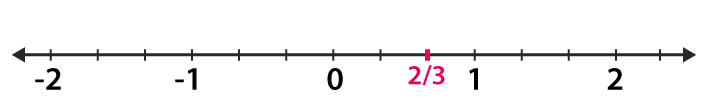(ii) We know that (3/4) is greater than 0 and less than 1.

∴ it lies between 0 and 1. It can be represented on number line as,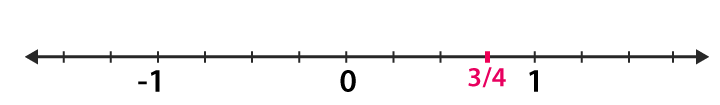(iii) We know that (3/8) is greater than 0 and less than 1.

∴ it lies between 0 and 1. It can be represented on number line as,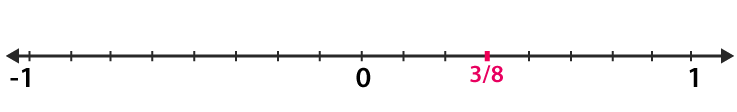(iv) We know that (-5/8) is greater than -1 and less than 0.

∴ it lies between 0 and -1. It can be represented on number line as,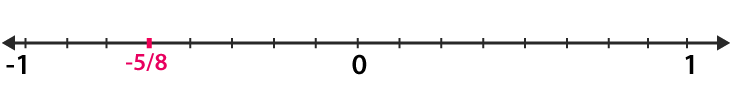(v) We know that (-3/16) is greater than -1 and less than 0.

∴ it lies between 0 and -1. It can be represented on number line as,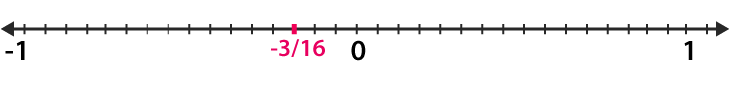(vi) We know that (-7/3) is greater than -3 and less than -2.

∴ it lies between -3 and -2. It can be represented on number line as,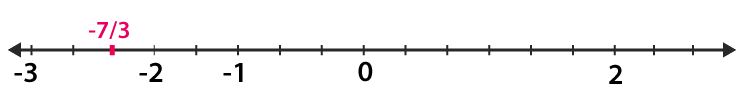(vii) We know that (22/-7) is greater than -4 and less than -3.

∴ it lies between -3 and -4. It can be represented on number line as,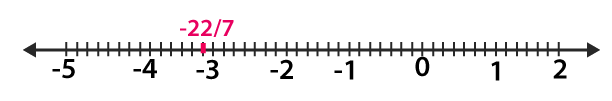(Viii) We know that (-31/3) is greater than -11 and less than -10.

∴ it lies between -10 and -11. It can be represented on number line as,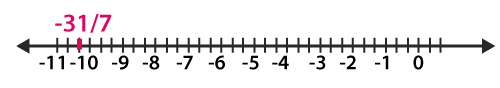2. Which of the two rational numbers in each of the following pairs of rational number is greater?
(i) (-3/8), 0

(ii) (5/2), 0

(iii) (– 4/11), (3/11)

(iv) (– 7/12), (5/- 8)

(v)  (4/-9), (– 3/- 7)

(vi) (– 5/8), (3/- 4)

(vii) (5/9), (-3/- 8)

(viii)  (5/- 8), (-7/12)

Solution:

(i) Given (-3/8), 0

We know that every positive rational number is greater than zero and every negative

rational number is smaller than zero. Thus, – (3/8) > 0

(ii)  Given (5/2), 0

We know that every positive rational number is greater than zero and every negative rational number is smaller than zero. Thus, (5/2) > 0

(iii) Given (– 4/11), (3/11)

We know that every positive rational number is greater than zero and every negative rational number is smaller than zero, also the denominator is same in given question now we have to compare the numerator, thus – 4/11 < 3/11.

(iv) Given (– 7/12), (5/- 8)

Consider (– 7/12)

Multiply both numerator and denominator by 2 then we get

(-7/12) × (2/2) = (-14/24)…… (1)

Now consider (5/-8)

Multiply both numerator and denominator by 3 we get

(5/-8) × (3/3) = (15/-24)…… (2)

The denominator is same in equation (1) and (2) now we have to compare the numerator, thus (– 7/12) > (5/- 8)

(v) Given (4/-9), (– 3/- 7)

Consider (4/-9)

Multiply both numerator and denominator by 7 then we get

(4/-9) × (7/7) = (28/-63)…… (1)

Now consider (-3/-7)

Multiply both numerator and denominator by 9 we get

(-3/-7) × (9/9) = (-27/-63)…… (2)

The denominator is same in equation (1) and (2) now we have to compare the numerator, thus (4/-9) < (– 3/- 7)

(vi) Given (– 5/8), (3/- 4)

Now consider (3/-4)

Multiply both numerator and denominator by 2 we get

(3/-4) × (2/2) = (6/-8)

The denominator is same in above equation now we have to compare the numerator, thus (– 5/8) > (3/- 4)

(vii) Given (5/9), (-3/- 8)

Consider (5/9)

Multiply both numerator and denominator by 8 then we get

(5/9) × (8/8) = (40/72)…… (1)

Now consider (5/-8)

Multiply both numerator and denominator by 9 we get

(-3/-8) × (9/9) = (-27/-72)…… (2)

The denominator is same in equation (1) and (2) now we have to compare the numerator, thus (5/9) > (-3/- 8)

(viii) Given (5/- 8), (-7/12)

Consider (5/-8)

Multiply both numerator and denominator by 3 then we get

(5/-8) × (3/3) = (15/-24)…… (1)

Now consider (-7/12)

Multiply both numerator and denominator by 2 we get

(-7/12) × (2/2) = (-14/24)…… (2)

The denominator is same in equation (1) and (2) now we have to compare the numerator, thus (5/- 8) < (-7/12)

3. Which of the two rational numbers in each of the following pairs of rational numbers is smaller?

(i) (-6/-13), (7/13)

(ii) (16/-5), 3

(iii) (-4/3), (8/-7)

(iv) (-12/5), (-3)

Solution:

(i) Given (-6/-13), (7/13)

Here denominator is same therefore compare the numerator,

Thus (-6/-13) < (7/13)

(ii) Given (16/-5), 3

We know that 3 is a whole number with positive sign

Therefore (16/-5) < 3

(iii) Given (-4/3), (8/-7)

Consider (-4/3)

Multiply both numerator and denominator by 7 then we get

(-4/3) × (7/7) = (-28/21)…… (1)

Now consider (8/-7)

Multiply both numerator and denominator by 3 we get

(8/-7) × (3/3) = (-24/21)…… (2)

The denominator is same in equation (1) and (2) now we have to compare the numerator, thus (-4/3) < (8/-7)

(iv) Given (-12/5), (-3)

Now consider (-3/1)

Multiply both numerator and denominator by 5 we get

(-3/1) × (5/5) = (-15/5)

The denominator is same in above equation, now we have to compare the numerator, thus (-12/5) > (-3)

4. Fill in the blanks by the correct symbol out of >, =, or <:

(i) (-6/7) …. (7/13)

(ii) (-3/5) …. (-5/6)

(iii) (-2/3) …. (5/-8)

(iv) 0 …. (-2/5)

Solution:

(i) (- 6/7) < (7/13)

Explanation:

Because every positive number is greater than a negative number.

(ii) (-3/5) > (-5/6)

Explanation:

Consider (-3/5)

Multiply both numerator and denominator by 6 then we get

(-3/5) × (6/6) = (-18/30)…… (1)

Now consider (-5/6)

Multiply both numerator and denominator by 5 we get

(-5/6) × (5/5) = (-25/30)…… (2)

The denominator is same in equation (1) and (2) now we have to compare the numerator, thus (-3/5) > (-5/6)

(iii) (-2/3) < (5/-8)

Explanation:

Consider (-2/3)

Multiply both numerator and denominator by 8 then we get

(-2/3) × (8/8) = (-16/24)…… (1)

Now consider (5/-8)

Multiply both numerator and denominator by 3 we get

(5/-8) × (3/3) = (15/-24)…… (2)

The denominator is same in equation (1) and (2) now we have to compare the numerator, thus (-2/3) < (5/-8)

(iv) 0 > (-2/5)

Explanation:

Because every positive number is greater than a negative number

5. Arrange the following rational numbers in ascending order:

(i) (3/5), (-17/-30), (8/-15), (-7/10)

(ii) (-4/9), (5/-12), (7/-18), (2/-3)

Solution:

(i) Given (3/5), (-17/-30), (8/-15), (-7/10)

The LCM of 5, 30, 15 and 10 is 30

Multiplying the numerators and denominators to get the denominator equal to the LCM i.e. 30

Consider (3/5)

Multiply both numerator and denominator by 6, then we get

(3/5) × (6/6) = (18/30) ….. (1)

Consider (8/-15)

Multiply both numerator and denominator by 2, then we get

(8/-15) × (2/2) = (16/-30) ….. (2)

Consider (-7/10)

Multiply both numerator and denominator by 3, then we get

(-7/10) × (3/3) = (-21/30) ….. (3)

In the above equation, denominators are same

Now on comparing the ascending order is:

(-7/10) < (8/-15) < (-17/30) < (3/5)

(ii) Given (-4/9), (5/-12), (7/-18), (2/-3)

The LCM of 9, 12, 18 and 3 is 36

Multiplying the numerators and denominators to get the denominator equal to the LCM i.e. 36

Consider (-4/9)

Multiply both numerator and denominator by 4, then we get

(-4/9) × (4/4) = (-16/36) ….. (1)

Consider (5/-12)

Multiply both numerator and denominator by 3, then we get

(5/-12) × (3/3) = (15/-36) ….. (2)

Consider (7/-18)

Multiply both numerator and denominator by 2, then we get

(7/-18) × (2/2) = (14/-36) ….. (3)

Consider (2/-3)

Multiply both numerator and denominator by 12, then we get

(2/-3) × (12/12) = (24/-36) ….. (4)

In the above equation, denominators are same

Now on comparing the ascending order is:

(2/-3) < ((-4/9) < (5/-12) < (7/-18)

6. Arrange the following rational numbers in descending order:

(i) (7/8), (64/16), (39/-12), (5/-4), (140/28)

(ii) (-3/10), (17/-30), (7/-15), (-11/20)

Solution:

(i) Given (7/8), (64/16), (39/-12), (5/-4), (140/28)

The LCM of 8, 16, 12, 4 and 28 is 336

Multiplying the numerators and denominators to get the denominator equal to the LCM i.e. 336

Consider (7/8)

Multiply both numerator and denominator by 42, then we get

(7/8) × (42/42) = (294/336) ….. (1)

Consider (64/16)

Multiply both numerator and denominator by 21, then we get

(64/16) × (21/21) = (1344/336) ….. (2)

Consider (39/-12)

Multiply both numerator and denominator by 28, then we get

(39/-12) × (28/28) = (-1008/336) ….. (3)

Consider (5/-4)

Multiply both numerator and denominator by 84, then we get

(5/-4) × (84/84) = (-420/336) ….. (4)

In the above equation, denominators are same

Now on comparing the descending order is:

(140/28) > (64/16) > (7/8) > (5/-4) > (36/-12)

(ii) Given (-3/10), (17/-30), (7/-15), (-11/20)

The LCM of 10, 30, 15 and 20 is 60

Multiplying the numerators and denominators to get the denominator equal to the LCM i.e. 60

Consider (-3/10)

Multiply both numerator and denominator by 6, then we get

(-3/10) × (6/6) = (-18/60) ….. (1)

Consider (17/-30)

Multiply both numerator and denominator by 2, then we get

(17/-30) × (2/2) = (34/-60) ….. (2)

Consider (7/-15)

Multiply both numerator and denominator by 4, then we get

(7/-15) × (4/4) = (28/-60) ….. (3)

In the above equation, denominators are same

Now on comparing the descending order is:

(-3/10) > (7/-15) > (-11/20) > (17/-30)

7. Which of the following statements are true:

(i) The rational number (29/23) lies to the left of zero on the number line.

(ii) The rational number (-12/-17) lies to the left of zero on the number line.

(iii) The rational number (3/4) lies to the right of zero on the number line.

(iv) The rational number (-12/-5) and (- 7/17) are on the opposite side of zero on the number line.

(v) The rational number (-2/15) and (7/-31) are on the opposite side of zero on the number line.

(vi) The rational number (- 3/-5) is on the right of (- 4/7) on the number line.

Solution:

(i) False

Explanation:

It lies to the right of zero because it is a positive number.

(ii) False

Explanation:

It lies to the right of zero because it is a positive number.

(iii) True

Explanation:

Always positive number lie on the right of zero

(iv) True

Explanation:

Because they are of opposite sign

(v) False

Explanation:

Because they both are of same sign

(vi) True

Explanation:

They both are of opposite signs and positive number is greater than the negative number. Thus, it is on the right of the negative number.